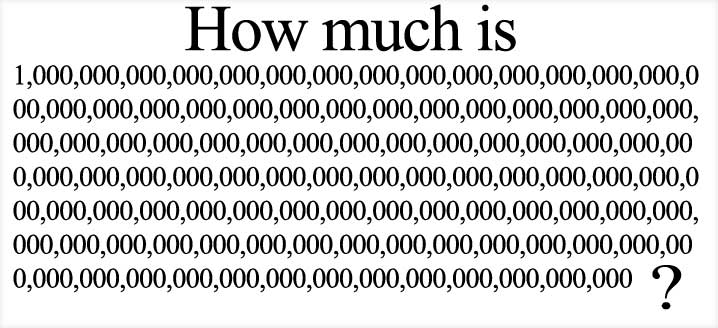# Pattern of an Odd Number

Putting an odd number of zeros between the following numbers below to form a new number, eleven is a factor of the new number.

29-38-47-56-65-74-83-92
How to test the claim
Let A be the front digit of those following numbers above.
Let B be the last digit of those following numbers above.
Add an odd number of zeros between A and B.
Eleven becomes the factor after adding an odd number of zeros beween
A and B.
If n=29 then A=2 and B=9
If n=38 then A=3 and B=8
If n=47 then A=4 and B=7
If n=56 then A=5 and B=6
If n=65 then A=6 and B=5
If n=74 then A=7 and B=4
If n=83 then A=8 and B=3
If n=92 then A=9 and B=2
Some pattern of odd zeros between A and B are listed below:
A0B -one zero between A and B
A000B-three zeros bettween A and B
A00000B-five zeros between A and B
A0000000B-seven zeros between A and B
A000000000B-nine zeros between A and B
A00000000000B- eleven zeros between A and B
Verify those arguments below:
If A0B is divided by eleven, the remainder is zero.
If A000B is divided by eleven, the remainder is zero.
If A00000B is divided by eleven, the remainder is zero.
If A0000000B is divided by eleven, the remainder is zero.
If A000000000B is divided by eleven, the remainder is zero.
If A00000000000B is divided by eleven, the remainder is zero.
Conceptual Ideas
A pattern of an odd number can be used to identify a number that can be factored with eleven. A number, that can be factored with eleven in that numerical triangle, should have an odd number of zeros between those two digits.

Background
An odd number is an integer of the form n=2k +1 , where k is an integer. If there exist a positive odd number of zeros between two digits that add to eleven in a number then the number can be factored with eleven.
_______________________________________________________________________
General formula to find a number N with an odd number zeros between A and B.
Let N be a number.
Let n be an integer greater or equal to 1.
N=(10^(2n))*A+B
If n is greater or equal to 1 then N=(10^(2n))*A+B is a number with odd number of zeros between A and B.
_________________________________________________________________________
Eight equations can be written with the general fromula:
1-N=(10^(2n))*2+9
2-N=(10^(2n))*3+8
3-N=(10^(2n))*4+7
4-N=(10^(2n))*5+6
5-N=(10^(2n))*6+5
6-N=(10^(2n))*7+4
7-N=(10^(2n))*8+3
8-N=(10^(2n))*9+2
_________________________________________________________________________
To find the factor, modify the N formula:
Let F be a number that is a factor of N.
Let n=0
Let k=1
F=(10^(2n))*A*K+B
Eight equations can be written to find the factor:
1-F=(10^(2n))*2*K+9
2-F=(10^(2n))*3*K+8
3-F=(10^(2n))*4*K+7
4-F=(10^(2n))*5*K+6
5-F=(10^(2n))*6*K+5
6-F=(10^(2n))*7*K+4
7-F=(10^(2n))*8*K+3
8-F=(10^(2n))*9*K+2
The value of F is eleven for all eight equations.
_________________________________________________________________________
Pattern of an Odd Number can be defined by this ratio:
[10^(2n))*A+B]/[(10^(2n))*A*K+B]
The numerator is defined for n greater or equal to 1.
The denominator is defined for for n=0 and k=1.
The remainder is zero.
we can write this relation below:
[10^(2n))*A+B]=0Mod[(10^(2n))*A*K+B]

_________________________________________________________________________
References

Weisstein, Eric W. “Odd Number.” From MathWorld–A Wolfram Web Resource.http://mathworld.wolfram.com/OddNumber.html